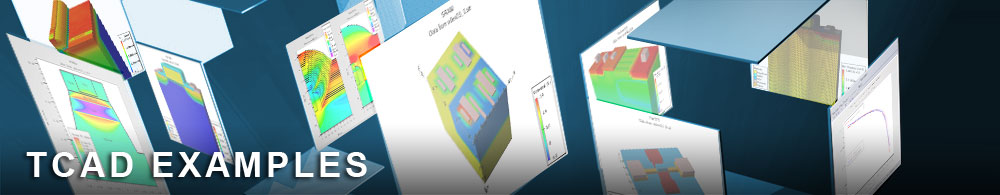Drift Diffusion mode space approach to cylindrical Surround Gate FET

## quantumex13.in : Drift Diffusion mode space approach to cylindrical Surround Gate FET

Requires: S-Pisces/Quantum
Minimum Versions: Atlas 5.28.1.R

This example demonstrates:

• Use of DD_MS model
• Quantum confinement
• Generation-recombination effects within DD_MS model
• Floating body effects

This example demonstrates the importance of quantum confinement and floating body effects in nanoscale surround gate FETs and shows how to use the Drift Diffusion mode space (DD_MS) model. The first part computes Id-Vg characteristics of an 4 nm in diameter SurFET without generation-recombination mechanisms. The second part switches on Shockly-Read-Hall recombination, band-to-band tunneling and impact ionization, and then compares the results.

Usage of DD_MS model is quite analogous to that of Schrodinger or mode-space NEGF (NEGF_MS) models. The DD_MS models is activated by an option DD_MS along with SCHRO (for electrons) and/or P.SCHRO (for holes) on the MODELS statement. The variable CARR on the METHOD statement should be set to zero, since multidimensional drift-diffusion solvers are not used. Due to a variation of electron density and potential in transverse direction, Dirichlet boundary conditions in contacts are not the best option. Instead, a quasi Fermi level is fixed, while electrostatic potential is subjected to von Neumann (zero electric field) boundary conditions, which are set by specifying REFLECT on the CONTACT statement.

The Schrodinger equation is solved in each transverse slice to find electron and/or hole eigen energies and wave functions. The minimum required number of eigen states is determined automatically, but may also be set by the parameter EIGEN on the MODELS statement. The DD_MS model is compatible with all Atlas Schrodinger solvers: 1D and cylindrical in Atlas2D and 2D in Atlas3D. In this example, the 1D Schrodinger equation is solved in cylindrical coordinates for all orbital quantum numbers at each slice in X direction. The eigen energies are then sorted together with the corresponding wave functions.

Position dependent eigen energies play a role of conduction (valence) band edge and are used to solve 1D drift-diffusion transport equations in each subband. The equations are discretized using Scharfetter-Gummel scheme. Atlas mobility models are fully integrated with the method and used in the discretization. DDMS always employs a rigorous mobility-diffusion relation, which is dimensionality dependent in case of Fermi-Dirac statistics.

Generation-recombination (G-R) mechanisms such as Shockley-Read-Hall, Auger and optical recombination, band-to-band tunneling, and impact ionization are available in the method. When generation-recombination mechanisms are present, it is possible to iterate between carrier density and G-R rates before solving the Poisson equation. The self-consistency between carrier density and G-R rates, achieved in this inner iteration procedure, will result in a more stable Poisson convergence. The number of inner iterations is controlled by the RGITER.DDMS=N and the RGCONV.DDMS=X parameters on the METHOD statement. Here, N is the maximum number of inner iterations (default is 0) and X is the minimum error between 0 and 1 (default is 1e-5). It is recommended that you first run a simulation without G-R mechanisms to get a feel for device behavior, and then increase G-R parameters.

In addition to a regular structure file, a detailed information on each subband can be stored in an extra log file by using option DDMS.LOG on SAVE or SOLVE statements. The file will contain subband-resolved quantities, such as eigen energies, carrier densities, current densities, quasi Fermi levels and generation-recombination rates.

Unlike the mode-space NEGF_MS model, the DD_MS model can handle only devices with uniform cross-section, because quantum mechanical coupling between electron subbands is neglected.

To load and run this example, select the Load button in DeckBuild > Examples. This will copy the input file and any support files to your current working directory. Select the Run button in DeckBuild to execute the example.